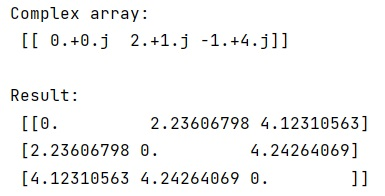# Calculate the Euclidean Distance Matrix using NumPy

In this tutorial, we will learn how to calculate the Euclidean distance matrix using Python NumPy? By Pranit Sharma Last updated : April 08, 2023

Suppose that we are given a set of points in 2-dimensional space and need to calculate the distance from each point to each other point.

## Efficiently calculating a Euclidean distance matrix

To calculate the Euclidean distance matrix using NumPy, we can take the advantage of the complex type. We will first create a complex array of our cells and we can then mesh the array so that we can have all the combinations finally we can get the distance by using the norm (difference of abs values from grid points).

Let us understand with the help of an example,

## Python Code to Calculate the Euclidean Distance Matrix using NumPy

```# Import numpy
import numpy as np

# Creating an array
arr = np.array([[0.+0.j, 2.+1.j, -1.+4.j]])

# Display complex array
print("Complex array:\n",arr,"\n")

# Mesh the array
m,n = np.meshgrid(arr, arr)

# Getting distance
res = abs(m-n)

# Display result
print("Result:\n",res,"\n")
```

### Output